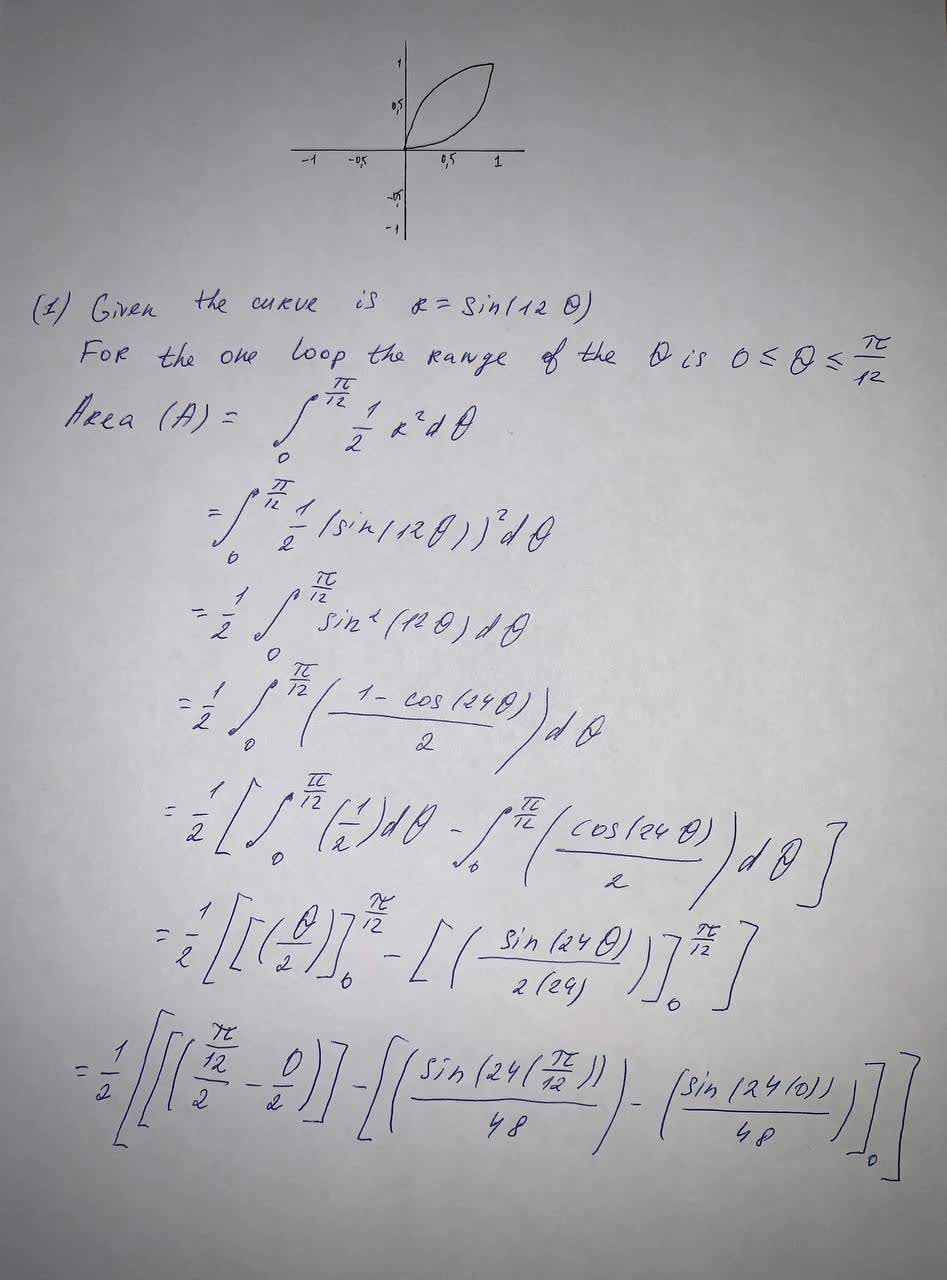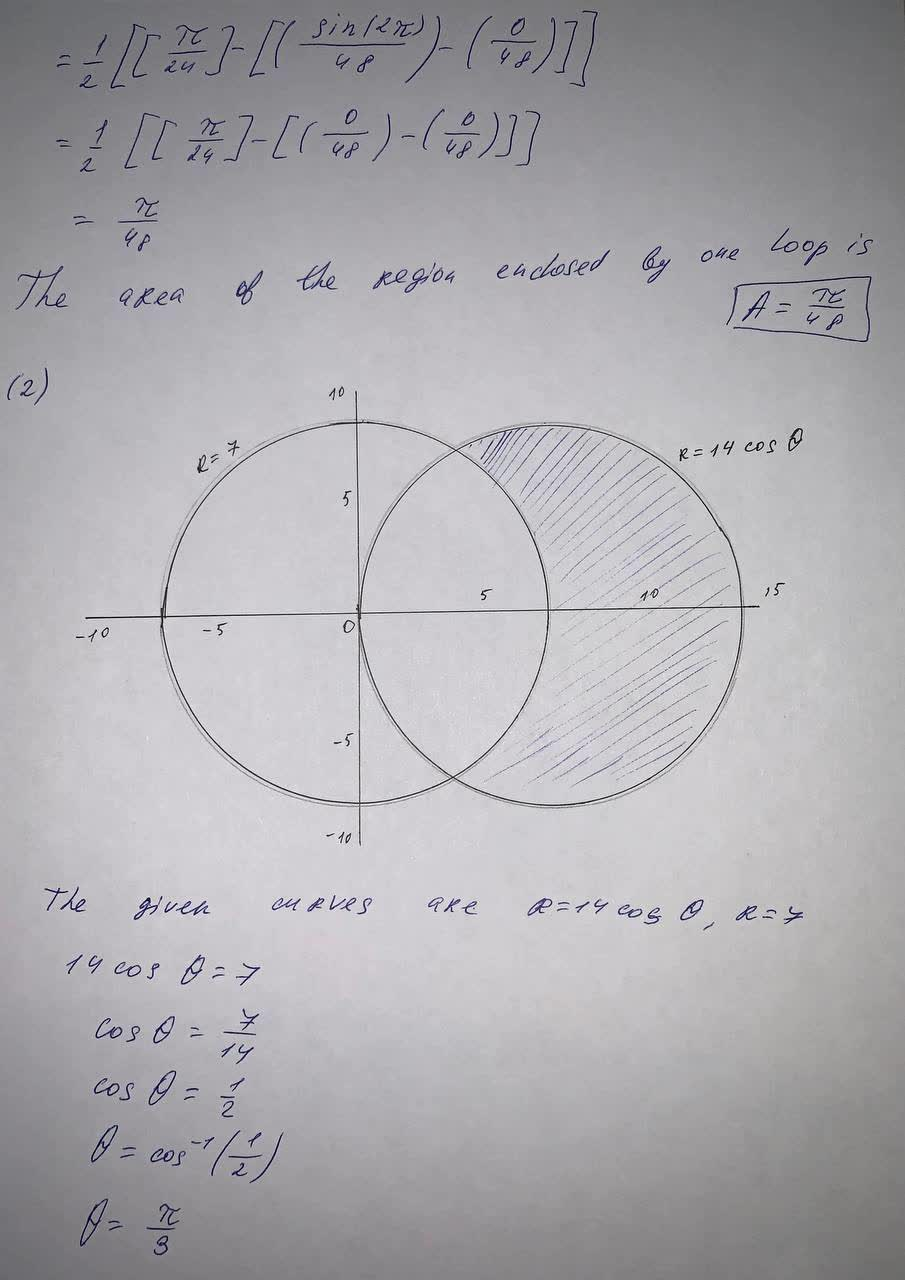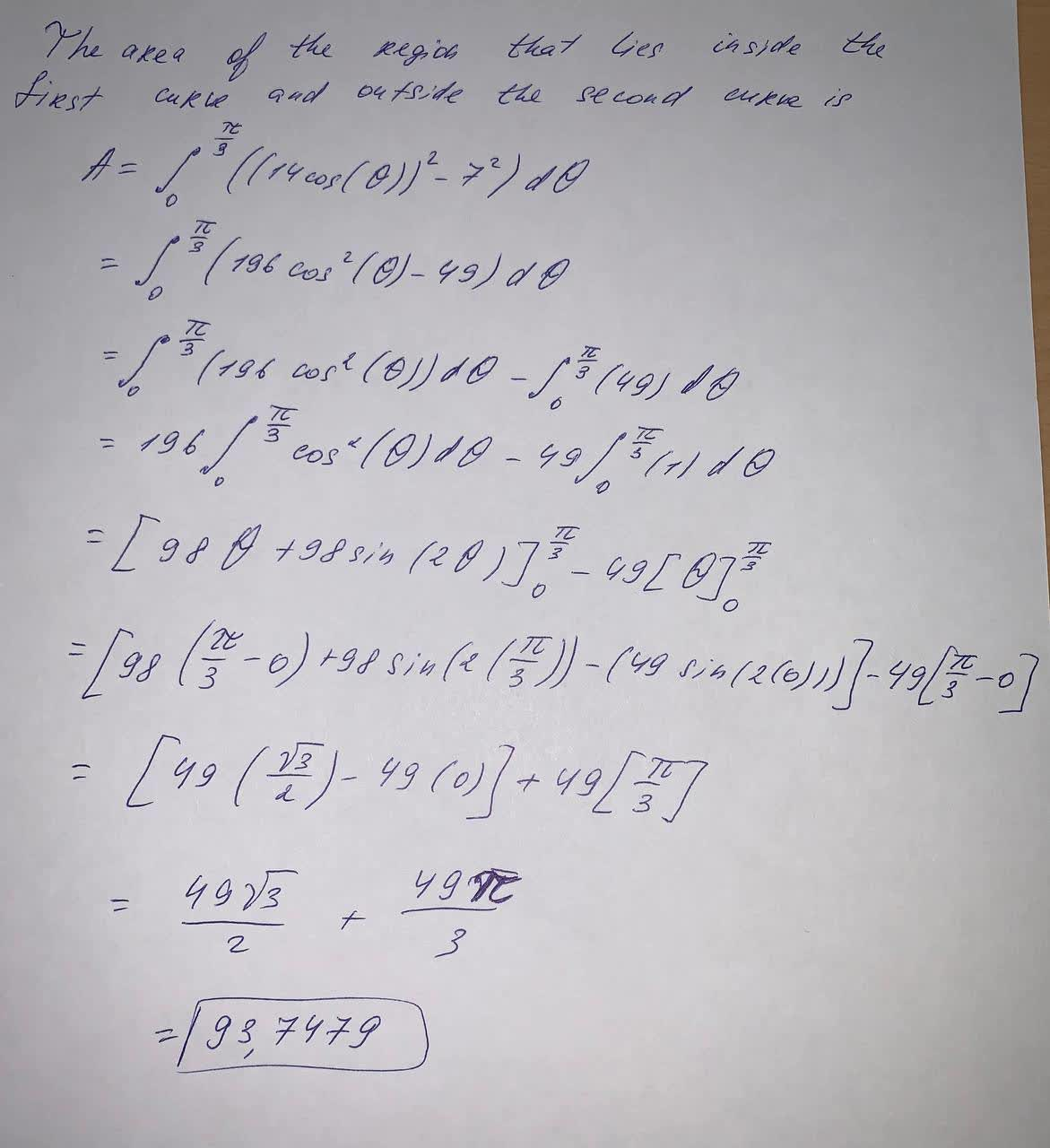Find the area of the region enclosed by one loop of the curve r=\sin 12 \theta Find the area of the region that lies inside the first curve outside the second curve. r=14 \cos \theta , r=7shadsiei 2021-05-12 Answered
Find the area of the region enclosed by one loop of the curve
$$r=\sin 12 \theta$$
Find the area of the region that lies inside the first curve outside the second curve.
$$r=14 \cos \theta , r=7$$

• Questions are typically answered in as fast as 30 minutes

Solve your problem for the price of one coffee

• Math expert for every subject
• Pay only if we can solve itinsonsipthinye
Hope this helps you: